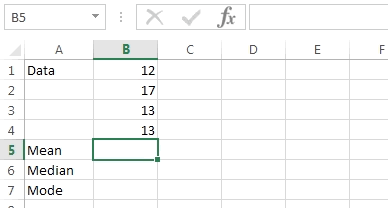### Excel formula

#### Summary Statistics (mean, mode, median) | # gomaths.net/4234         User submitted, thanks to Simon Job

Calculate summary statistics: mean, mode, median

Mean: `=AVERAGE(B1:B4)`

Mode: `=MODE(B1:B4)`

Median: `=MEDIAN(B1:B4)`

where `B1:B4` is the range of cells containing numerical data.